# Difference between revisions of "State Feedback"

 Prev: Linear Systems Chapter 6 - State Feedback Next: Output Feedback

This chapter describes how feedback can be used shape the local behavior of a system. The concept of reachability is introduced and used to investigate how to "design" the dynamics of a system through placement of its eigenvalues. In particular, it will be shown that under certain conditions it is possible to assign the system eigenvalues to arbitrary values by appropriate feedback of the system state.

## Textbook Contents

• 1. Introduction
• 2. Reachability
• 3. Stabilization by State Feedback
• 4. State Feedback Design Issues
• 5. Integral Action
• 8. Exercises

## Chapter Summary

This chapter describes how state feedback can be used to design the (closed loop) dynamics of the system:

1. A linear system with dynamicsis said to be reachable if we can find an inputdefined on the intervalthat can steer the system from a given final pointto a desired final point.

2. The reachability matrix for a linear system is given byA linear system is reachable if and only if the reachability matrix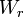is invertible (assuming a single intput/single output system). Systems that are not reachable have states that are constrained to have a fixed relationship with each other.

3. A linear system of the formis said to be in reachable canonical form. A system in this form is always reachable and has a characteristic polynomial given by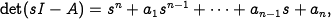A reachable linear system can be transformed into reachable canonical form through the use of a coordinate transformation.

4. A state feedback law has the form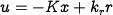whereis the reference value for the output. The closed loop dynamics for the system are given byThe stability of the system is determined by the stability of the matrix. The equilibrium point and steady state output (assuming the systems is stable) are given byChoosing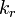asgives.

5. If a system is reachable, then there exists a feedback law of the formthe gives a closed loop system with an arbitrary characteristic polynomial. Hence the eigenvalues of a reachable linear system can be placed arbitrarily through the use of an appropriate feedback control law.

6. Integral feedback can be used to provide zero steady state error instead of careful calibration of the gain. An integral feedback controller has the form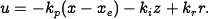whereis the integral error. The gains,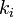andcan be found by designing a stabilizing state feedback for the system dynamics augmented by the integrator dynamics.

7. A linear quadratic regulator minimizes the cost functionThe solution to the LQR problem is given by a linear control law of the formwhereis a positive definite, symmetric matrix that satisfies the equationThis equation is called thealgebraic Riccati equation and can be solved numerically.